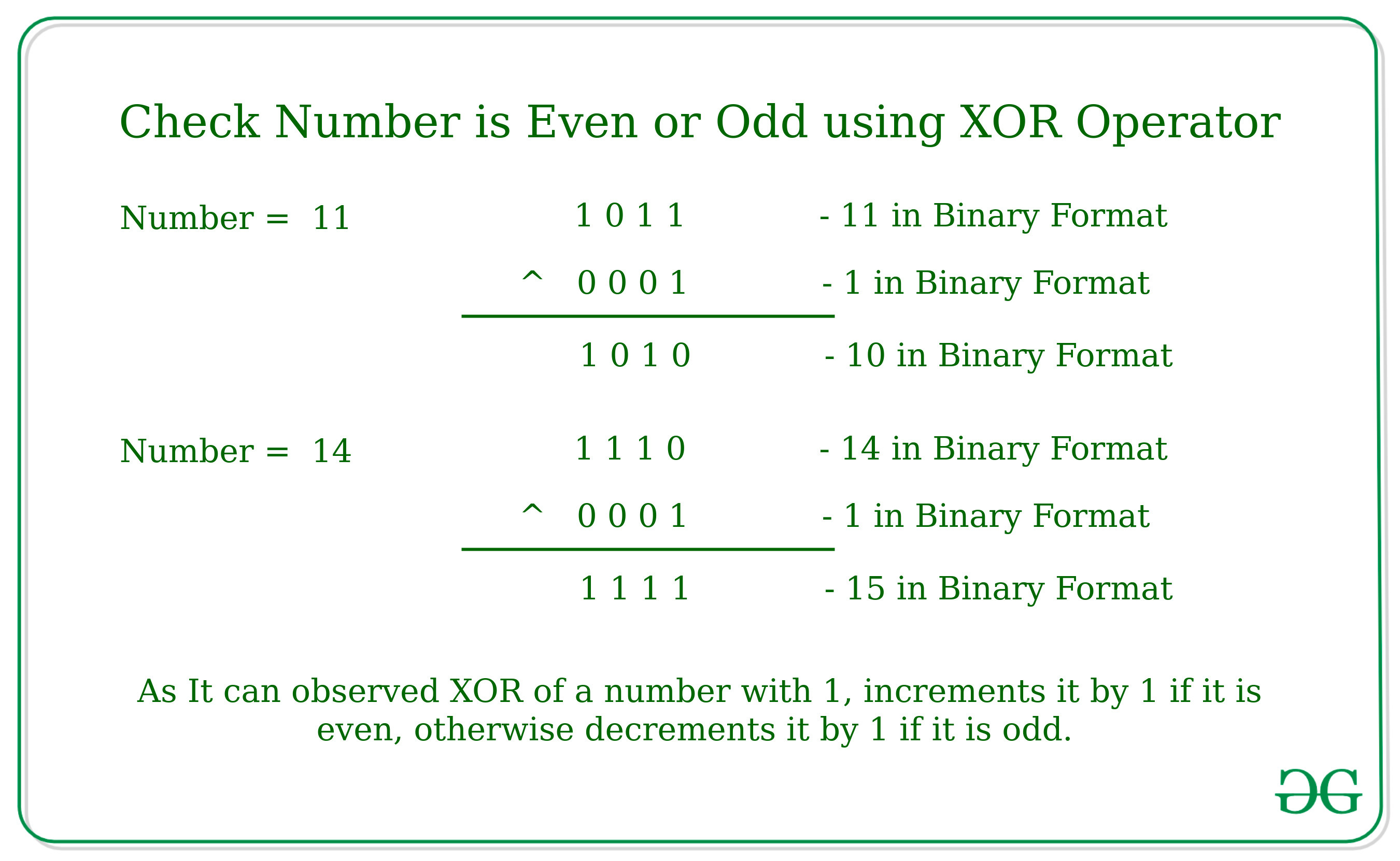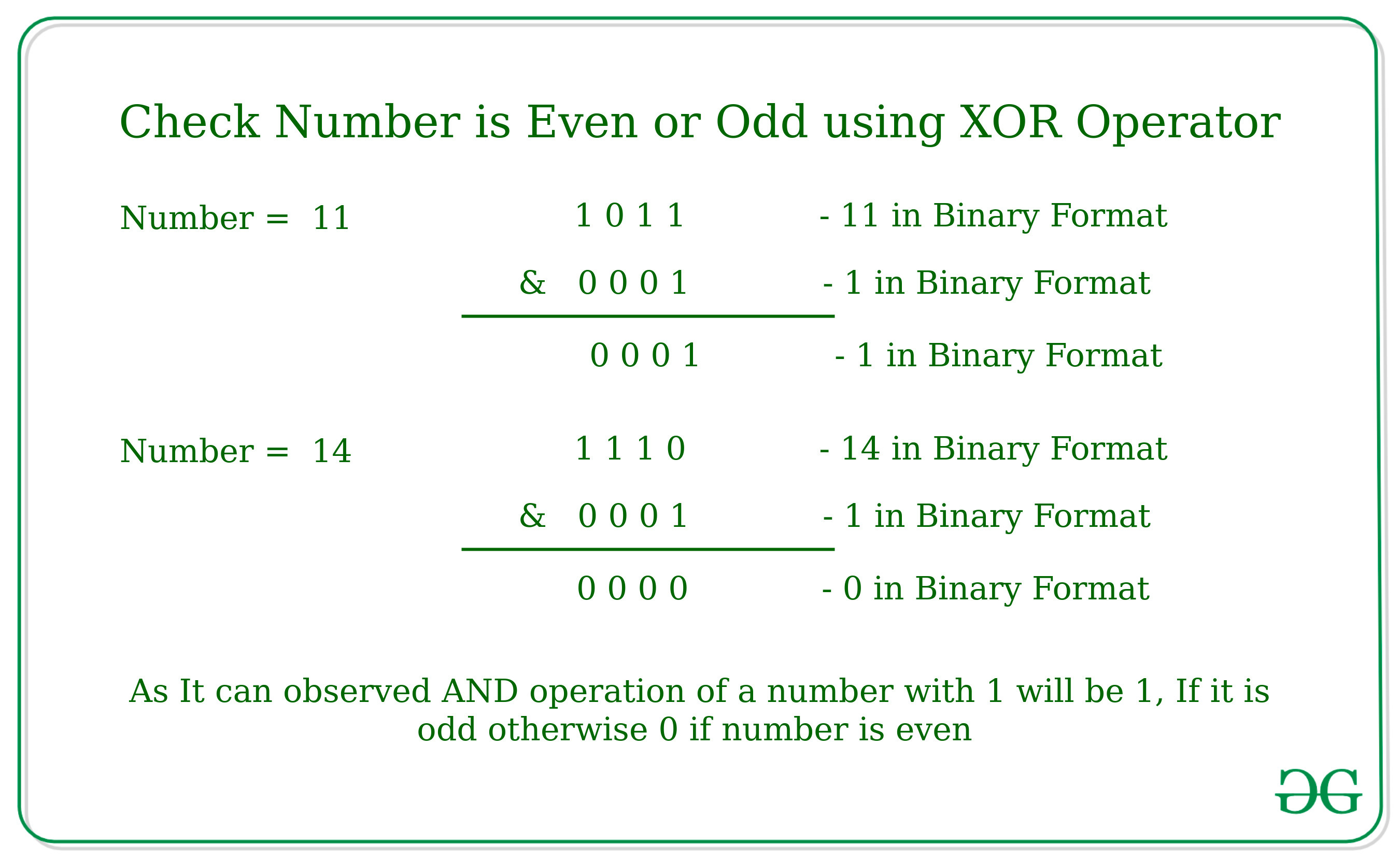Related Articles

# Check if a Number is Odd or Even using Bitwise Operators

• Difficulty Level : Basic
• Last Updated : 12 Apr, 2021

Given a number N, the task is to check whether the number is even or odd using Bitwise Operators.
Examples:

Input: N = 11
Output: Odd
Input: N = 10
Output: Even

Following Bitwise Operators can be used to check if a number is odd or even:

1. Using Bitwise XOR operator:
The idea is to check whether the last bit of the number is set or not. If the last bit is set then the number is odd, otherwise even.
As we know bitwise XOR Operation of the Number by 1 increment the value of the number by 1 if the number is even otherwise it decrements the value of the number by 1 if the value is odd.Below is the implementation of the above approach:

## C++

 `// C++ program to check for even or odd``// using Bitwise XOR operator` `#include ``using` `namespace` `std;` `// Returns true if n is even, else odd``bool` `isEven(``int` `n)``{` `    ``// n^1 is n+1, then even, else odd``    ``if` `(n ^ 1 == n + 1)``        ``return` `true``;``    ``else``        ``return` `false``;``}` `// Driver code``int` `main()``{``    ``int` `n = 100;``    ``isEven(n)``? cout << ``"Even"``: cout << ``"Odd"``;` `    ``return` `0;``}`

## Java

 `// Java program to check for even or odd``// using Bitwise XOR operator``class` `GFG``{` `    ``// Returns true if n is even, else odd``    ``static` `boolean` `isEven(``int` `n)``    ``{` `        ``// n^1 is n+1, then even, else odd``        ``if` `((n ^ ``1``) == n + ``1``)``            ``return` `true``;``        ``else``            ``return` `false``;``    ``}` `    ``// Driver code``    ``public` `static` `void` `main(String[] args)``    ``{``        ``int` `n = ``100``;``        ``System.out.print(isEven(n) == ``true` `? ``"Even"` `: ``"Odd"``);``    ``}``}` `// This code is contributed by Rajput-Ji`

## Python3

 `# Python3 program to check for even or odd``# using Bitwise XOR operator` `# Returns true if n is even, else odd``def` `isEven( n) :` `    ``# n^1 is n+1, then even, else odd``    ``if` `(n ^ ``1` `=``=` `n ``+` `1``) :``        ``return` `True``;``    ``else` `:``        ``return` `False``;` `# Driver code``if` `__name__ ``=``=` `"__main__"` `:``    ``n ``=` `100``;``    ``print``(``"Even"``) ``if` `isEven(n) ``else` `print``( ``"Odd"``);` `# This code is contributed by AnkitRai01`

## C#

 `// C# program to check for even or odd``// using Bitwise XOR operator``using` `System;` `class` `GFG``{` `    ``// Returns true if n is even, else odd``    ``static` `bool` `isEven(``int` `n)``    ``{` `        ``// n^1 is n+1, then even, else odd``        ``if` `((n ^ 1) == n + 1)``            ``return` `true``;``        ``else``            ``return` `false``;``    ``}` `    ``// Driver code``    ``public` `static` `void` `Main(String[] args)``    ``{``        ``int` `n = 100;``        ``Console.Write(isEven(n) == ``true` `? ``"Even"` `: ``"Odd"``);``    ``}``}` `// This code is contributed by Rajput-Ji`

## Javascript

 ``
Output
`Even`

2. Using Bitwise AND operator:
The idea is to check whether the last bit of the number is set or not. If last bit is set then the number is odd, otherwise even.
As we know bitwise AND Operation of the Number by 1 will be 1, If it is odd because the last bit will be already set. Otherwise it will give 0 as output.Below is the implementation of the above approach:

## C++

 `// C++ program to check for even or odd``// using Bitwise AND operator``#include ``using` `namespace` `std;``  ` `// Returns true if n is even, else odd``bool` `isEven(``int` `n)``{``    ``// n&1 is 1, then odd, else even``    ``return` `(!(n & 1));``}``  ` `// Driver code``int` `main()``{``    ``int` `n = 101;``    ``isEven(n)``    ``? cout << ``"Even"``    ``: cout << ``"Odd"``;``    ``return` `0;``}`

## Java

 `// Java program to check for even or odd``// using Bitwise AND operator``class` `GFG``{``    ` `// Returns true if n is even, else odd``static` `boolean` `isEven(``int` `n)``{``    ``// n&1 is 1, then odd, else even``    ``return` `((n & ``1``)!=``1``);``}``    ` `// Driver code``public` `static` `void` `main(String[] args)``{``    ``int` `n = ``101``;``    ``System.out.print(isEven(n) == ``true` `? ``"Even"` `: ``"Odd"``);``}``}` `// This code is contributed by PrinciRaj1992`

## Python3

 `# Python3 program to check for even or odd``# using Bitwise AND operator` `# Returns true if n is even, else odd``def` `isEven(n) :` `    ``# n&1 is 1, then odd, else even``    ``return` `(``not``(n & ``1``));` `# Driver code``if` `__name__ ``=``=` `"__main__"` `:` `    ``n ``=` `101``;``    ``if` `isEven(n) :``        ``print``(``"Even"``)``    ``else``:``        ``print``(``"Odd"``);` `# This code is contributed by AnkitRai01`

## C#

 `// C# program to check for even or odd``// using Bitwise AND operator``using` `System;` `class` `GFG``{``    ` `    ``// Returns true if n is even, else odd``    ``static` `bool` `isEven(``int` `n)``    ``{``        ``// n&1 is 1, then odd, else even``        ``return` `((n & 1) != 1);``    ``}``        ` `    ``// Driver code``    ``public` `static` `void` `Main()``    ``{``        ``int` `n = 101;``        ``Console.Write(isEven(n) == ``true` `? ``"Even"` `: ``"Odd"``);``    ``}``}` `// This code is contributed by AnkitRai01`

## Javascript

 ``
Output
`Odd`

3. Using Bitwise OR operator: The idea is to check whether the last bit of the number is set or not. If the last bit is set then the number is odd, otherwise even. As we know bitwise OR Operation of the Number by 1 increment the value of the number by 1 if the number is even otherwise it will remain unchanged. So, if after OR operation of number with 1 gives a result which is greater than the number then it is even and we will return true otherwise it is odd and we will return false.

Below is the implementation of the above approach:

## C++

 `// C++ program to check for even or odd``// using Bitwise OR operator` `#include ``using` `namespace` `std;` `// Returns true if n is even, else odd``bool` `isEven(``int` `n)``{` `    ``// n|1 is greater than n, then even, else odd``    ``if` `((n | 1) > n)``        ``return` `true``;``    ``else``        ``return` `false``;``}` `// Driver code``int` `main()``{``    ``int` `n = 100;``    ``isEven(n)``    ``? cout << ``"Even"``    ``: cout << ``"Odd"``;` `    ``return` `0;``}`

## Java

 `// Java program to check for even or odd``// using Bitwise OR operator``class` `GFG``{``    ``// Returns true if n is even, else odd``    ``static` `boolean` `isEven(``int` `n)``    ``{` `        ``// n|1 is greater than n, then even, else odd``        ``if` `((n | ``1``) > n)``            ``return` `true``;``        ``else``            ``return` `false``;``    ``}` `    ``// Driver code``    ``public` `static` `void` `main(String[] args)``    ``{``        ``int` `n = ``100``;``        ``System.out.print(isEven(n) == ``true` `? ``"Even"` `: ``"Odd"``);``    ``}``}`

## Python3

 `# Python3 program to check for even or odd``# using Bitwise OR operator` `# Returns true if n is even, else odd``def` `isEven( n) :` `    ``# n|1 is greater then n, then even, else odd``    ``if` `(n | ``1` `> n) :``        ``return` `True``;``    ``else` `:``        ``return` `False``;` `# Driver code``if` `__name__ ``=``=` `"__main__"` `:``    ``n ``=` `100``;``    ``print``(``"Even"``) ``if` `isEven(n) ``else` `print``( ``"Odd"``);`

## C#

 `// C# program to check for even or odd``// using Bitwise XOR operator``using` `System;` `class` `GFG``{` `    ``// Returns true if n is even, else odd``    ``static` `bool` `isEven(``int` `n)``    ``{` `        ``// n|1 is greater then n, then even, else odd``        ``if` `((n | 1) > n)``            ``return` `true``;``        ``else``            ``return` `false``;``    ``}` `    ``// Driver code``    ``public` `static` `void` `Main(String[] args)``    ``{``        ``int` `n = 100;``        ``Console.Write(isEven(n) == ``true` `? ``"Even"` `: ``"Odd"``);``    ``}``}` `// This code is contributed by Rajput-Ji`

## Javascript

 ``
Output
`Even`

Time Complexity: O(1)

Attention reader! All those who say programming isn’t for kids, just haven’t met the right mentors yet. Join the  Demo Class for First Step to Coding Coursespecifically designed for students of class 8 to 12.

The students will get to learn more about the world of programming in these free classes which will definitely help them in making a wise career choice in the future.

My Personal Notes arrow_drop_up